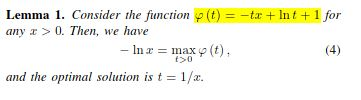# Illegal operation: {concave} - {complex affine}

Hi all

``````clc;clear ;close all;

% Simulation parameters
M        = 8 ;      % # of BS antenna
N        = 2 ;      % # of User antennas
K        = 4;       % # of Users
eta      = 0.8;     % energy conversion coefficient
sigma2   = 1 ;      % The noise covariance at the ID receiver(watt)
%epsilon = 3 ;      % pathloss exponent
RT       = 4 ;      % The target transmission rate(Mbps)
Bs       = 10;      %The bandwidth is 100 KHz
QOS      = RT/Bs;   %0.4(Mbps/Hz)
Pth_dBm  = 46;      %transit power threshold(dBm)
Pth      = 10^(Pth_dBm/10)/1000;%mw
alpha    = rand(1,K);% power splitting ratio
p        = (Pth/K)*ones(1,K); % power splitting ratio

%%XXXXXXXXXXXXXXXXXXXXXXXXXXXXXXXXXXXXXXXXXXXXXXXXXXXXXXXXXXXXXXXXXX
%% Simulation
%% Modeling the Rayleigh fading channel(N*M)
num_ch=K*N;
H=randn(num_ch,M)+1i*randn(num_ch,M);

W_0=zeros(N,N,K);%initial W matrix
for ss=1:K
W_0(:,:,ss)=randn(N,N)+1i*randn(N,N);
end
thrsh=0.001; %the threshold for while loop stopping criteria.
diff=10;

while diff>=thrsh

% t calculation step.
t_j=zeros(1,K);
for x=1:K
Hj=H((x-1)*N+1:(x-1)*N+N,:);
Wj=W_0(:,:,x);
int_user=setdiff(1:K,x);
power_int=sum(p(int_user));
temp=(alpha(x)*power_int*trace(Hj'*Wj*Hj))^(-1)
t_j(1,x)=temp;
end

% cvx version for problem W
cvx_begin
variable W1(N,N) complex symmetric

[numel_SINR,denumel_SINR,cost]=Rate_m3(K,H,N,W1,t_j,alpha,sigma2,p);

maximize(cost)
subject to

for m=1:K
numel_SINR(1,m)-(2^QOS-1)*denumel_SINR(1,m)>=0
end

cvx_end
end
###########################

function[numel_SINR,denumel_SINR,cost]=Rate_m3(K,H,N,W1,t_j,alpha,sigma2,p)

cost=0;
numel_SINR=zeros(1,K);
denumel_SINR=zeros(1,K);

d=1;
Hj=H((d-1)*N+1:(d-1)*N+N,:);
pi_numel=setdiff(1:K,d);
alf=alpha(d);

Tmp=log_det(0.5*((alf*sum(p)*trace(Hj'*W1*Hj)+sigma2)+(alf*sum(p)*trace(Hj'*W1*Hj)+sigma2)'))-t_j(d)*alf*sum(p(pi_numel))*real(trace(Hj'*W1*Hj))+log_det(0.5*(t_j(d)+t_j(d)'))+1;
cost=cost+Tmp;
numel_SINR(1,d)=p(d)*alf*trace(Hj'*W1*Hj);
denumel_SINR(1,d)=alf*sum(p(pi_numel))*trace(Hj'*W1*Hj)+sigma2;

end``````
1 Like

` -t_j(d)*alf*sum(p(pi_numel))` is a complex scalar.

` real(trace(Hj'*W1*Hj))` is a real affine scalar.

Hence their product is a complex affine scalar. Hence the error message in the line
`Tmp=log_det(0.5*((alf*sum(p)*trace(Hj'*W1*Hj)+sigma2)+(alf*sum(p)*trace(Hj'*W1*Hj)+sigma2)'))-t_j(d)*alf*sum(p(pi_numel))*real(trace(Hj'*W1*Hj))+log_det(0.5*(t_j(d)+t_j(d)'))+1;`

I have no idea what you’re trying to do, so I’ll leave the fix to you. Perhaps you need to apply `real(...)` differently.

Thank you Mr. Mark for your attention.This is my problem …

We have a W for each user And we want to optimize it for every user. We used variable change to solve the problem. W can be a complex number or a vector. But we always get the same error.

I have no idea what any of that means.

Is the left-hand side of
`numel_SINR(1,m)-(2^QOS-1)*denumel_SINR(1,m) >= 0`
supposed to evaluate to a real scalar? Forget CVX for the moment and just pretend this is a MATLAB expression. Choose arbitrary complex values of `W1`. Does the ;left-hand side evaluate to a real scalar? If not, there is something wrong with your model, unless you want the real and imaginary parts to each be `>= 0`, in which case you can enter those as separate constraints by using `real` and `imag`.

In fact, this is my main problem. And I used a lem to solve it for the variable W j.

I have already proved that the problem is concave with respect to my variables. But I got the same errors from CVX.

The lem I usedHave you proved the relevant quantities are real? ifnot, they can’t be convex.

Have you carried out the exercise I suggested in my previous post?